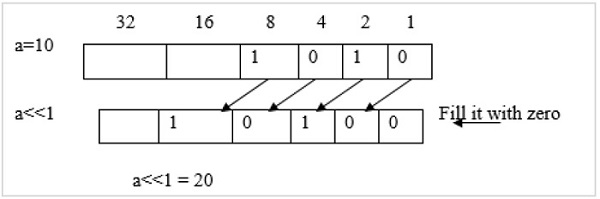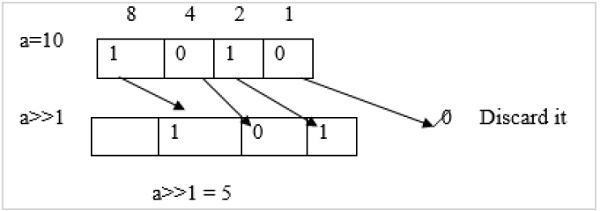# What are the shift operations in C language?

CServer Side ProgrammingProgramming

## Problem

What is the simple program to show the left, right shifts, and complement of a number by using C language?

## Solution

### Left Shift

If the value of a variable is left-shifted one time, then its value gets doubled.

For example, a = 10, then a<<1 = 20### Right shift

If the value of a variable is right-shifted one time, then its value becomes half the original value.

For example, a = 10, then a>>1 = 5## Example

Following is the C program for the shift operations −

Live Demo

#include<stdio.h>
main (){
int a=9;
printf("Rightshift of a = %d\n",a>>1);//4//
printf("Leftshift of a = %d\n",a<<1);//18//
printf("Compliment of a = %d\n",~a);//-[9+1]//
printf("Rightshift by 2 of a = %d\n",a>>2);//2//
printf("Leftshift by 2 of a = %d\n",a<<2);//36//
}

## Output

When the above program is executed, it produces the following result −

Rightshift of a = 4
Leftshift of a = 18
Compliment of a = -10
Rightshift by 2 of a = 2
Leftshift by 2 of a = 36